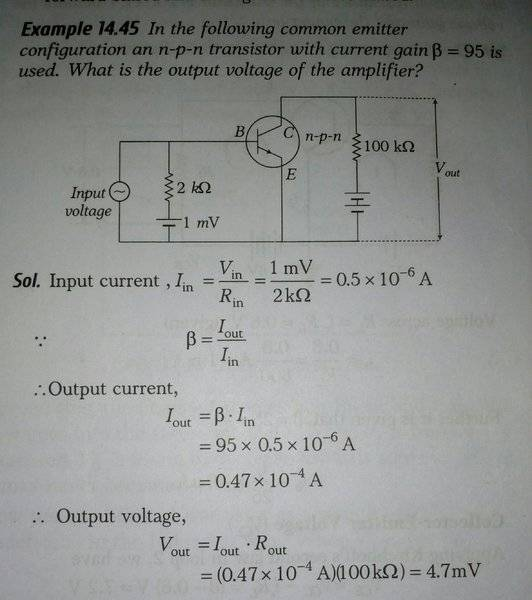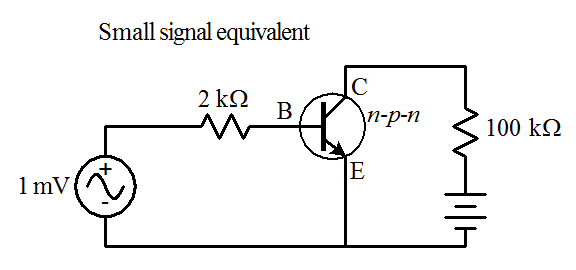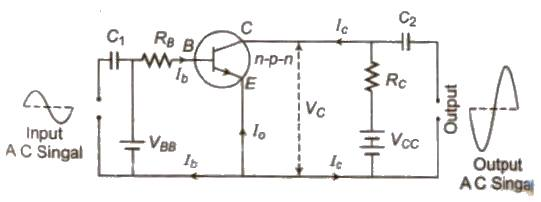# Transistor : Output voltage of the amplifier

• Jahnavi
Jahnavi

## Homework Statement## The Attempt at a Solution

This is a solved example given in my Physics textbook . I am not understanding a couple of things .

1) How is Iin calculated as Vin/Rin ?

Why is the book not taking potential difference between Base Emitter into consideration ?

Instead shouldn't Kirchoff's Voltage rule be applied in the Base Emitter loop ?

I think it should be Iin =( 1mV -VBE )/2KΩ

2) Shouldn't the diagram be showing Vout across 100kΩ ?

I don't understand why book considers Vout to be VCE ?

#### Attachments

Homework Helper
Gold Member
##V_{BE}## is equal to input voltage which probably is small, much smaller than 1mV, so ##1mV-V_{BE}\approx1mV##.

Jahnavi
##V_{BE}## is equal to input voltage which probably is small, much smaller than 1mV, so ##1mV-V_{BE}\approx1mV##.

I don't think this is the case .

I think in a silicon PN junction potential difference between Base and Emitter is about 0.7 V .

This is quite large as compared to the bias battery .

Homework Helper
Gold Member
I don't think this is the case .

I think in a silicon PN junction potential difference between Base and Emitter is about 0.7 V .

This is quite large as compared to the bias battery .
You might be right , I am not an EE and don't know much about transistors, just said to share my thought on this.

Jahnavi
Homework Helper
Gold Member
To me, it looks like a problem that is poorly presented. e.g., to forward bias ## V_{BE} ## , you normally need ## V_{BE}=.7 ## volts. They also don't specify the collector bias voltage. Perhaps someone else has a solution, but my recommendation is to not spend much time on this one as is. This "common emitter" electronic configuration is a good one to know, but this problem looks to me to be a poor one to use to try to learn it.

Last edited:
•Jahnavi
Jahnavi
Thanks .

Suppose instead of 1mV battery there is a 1 V battery , then do you think what I did in the original post Iin=( 1V -VBE)/2KΩ is correct ?

Homework Helper
Gold Member
Thanks .

Suppose instead of 1mV battery there is a 1 V battery , then do you think what I did in the original post Iin=( 1V -VBE)/2KΩ is correct ?
I looked at the problem in the "link" you had posted. For that one, with the book's example, a ## V_{BE} ## of 18.5 Volts I think is totally inaccurate. I expect if you hooked it up in the lab, you might find that ## I_B ## would be larger than what is computed using the value ## \beta=200 ##, and you would find ## V_{BE}=.7 ## volts. And then I think you might have removed the "link": https://www.physicsforums.com/threads/find-the-state-of-transistor.935500/#post-5909999 ## \\ ## Edit: And on this one. a base voltage of 1 volt might work, but they still need to specify the collector voltage. Also ## V_{in} ## (ac) usually gets coupled in with a capacitor, and ## V_{out} ## (ac) is usually also coupled with a capacitor.

•Jahnavi and cnh1995
Jahnavi
Thank you .

Do you think post 7 is correct ?

Homework Helper
Gold Member
Thank you .

Do you think post 7 is correct ?
That part works, but please see my edited additions to post 8.

Homework Helper
Gold Member
Thanks .

Suppose instead of 1mV battery there is a 1 V battery , then do you think what I did in the original post Iin=( 1V -VBE)/2KΩ is correct ?
I don't think this problem is practical and as Charles says, not much helpful in learning CE configuration.
The battery needs to be in series with the input ac source (theoretically). In this circuit, as per circuit theory, the battery can't dc-bias the transistor as the ac source is directly connected across the BE junction. Even if the battery were a 10V one, it couldn't dc-bias the transistor in this diagram.

•Homework Helper
Gold Member
I don't think this problem is practical and as Charles says, not much helpful in learning CE configuration.
The battery needs to be in series with the input ac source (theoretically). In this circuit, as per circuit theory, the battery can't dc-bias the transistor as the ac source is directly connected across the BE junction. Even if the battery were a 10V one, it couldn't dc-bias the transistor in this diagram.
See also my post 8. IMO, this textbook looks like it may contain numerous errors. I encountered that type of thing from time to time in my college days. The best solution would be for the OP @Jahnavi to learn the material from a book that presents it better and with fewer mistakes, if that is at all possible.

Jahnavi
The battery needs to be in series with the input ac source (theoretically).

@Charles Link , do you also think this is correct ?

Homework Helper
Gold Member
See also my post 8. IMO, this textbook looks like it may contain numerous errors. I encountered that type of thing from time to time in my college days. The best solution would be for the OP @Jahnavi to learn the material from a book that presents it better and with fewer mistakes, if that is at all possible.
I have seen many junior college physics books in India showing wrong biasing circuit for CE amplifier. @Jahnavi, I believe that includes the NCERT textbook as well.
I agree that you should study amplifiers from some standard electronis books (or COP by H C Verma).

•Homework Helper
Gold Member
@Charles Link , do you also think this is correct ?
The way they show it, if you use ## V=1 ## volt, it would work, provided the ## V_{in} ## was ac coupled (with a capacitor).

Homework Helper
Gold Member
The way they show it, if you use ## V=1 ## volt would work, provided the ## V_{in} ## was ac coupled (with a capacitor).
Yes, but I wasn't including a coulping capacitor in my reasoning. If there isn't one, the battery is ineffective.

•Jahnavi
The way they show it, if you use ## V=1 ## volt, it would work, provided the ## V_{in} ## was ac coupled (with a capacitor).

But then it would be something like an input AC signal placed parallel to a DC battery (1 V)

What would then be the net effective voltage applied across Base Emitter ?

This is so confusing.

Homework Helper
Gold Member
then do you think what I did in the original post Iin=( 1V -VBE)/2KΩ is correct ?
That Iin wouldn't be the true Iin as it won't flow through the base. It only flows through the battery. The base current will be determined by the input ac voltage, which will be the same even if you remove the battery.

Jahnavi
That Iin wouldn't be the true Iin as it won't flow through the base. It only flows through the battery. The base current will be determined by the input ac voltage, which will be the same even if you remove the battery.

I was assuming AC input signal was not present .

Homework Helper
Gold Member
But then it would be something like an input AC signal placed parallel to a DC battery (1 V)
The net BE voltage will be the input ac voltage. The battery is redundant as far as dc biasing is concerned.
Edit: assuming no coupling capacitor.

I was assuming AC input signal was not present .
Ok, but when you connect the ac source, Iin≠IB.

Homework Helper
Gold Member
Usually coupling capacitors are used on the ac signals, so that the bias voltage would not be short-circuited by the ac input connection. Meanwhile, oftentimes the ac signal can be a very small signal, sometimes from sensitive sensors where the signal needs to be amplified. A ## V_{DC}=1 ## volt could destroy some sensors. The ac coupling with a capacitor is quite necessary in these amplifiers.

•cnh1995
Mentor
I suspect that the given circuit diagram is flawed; Perhaps the original intention was to represent the bias network's essential small-signal equivalent circuit, but something went wrong after the illustrator got hold of it. The 1mV apparently should be associated with the input source, not a base bias battery. Or, that battery originally was meant to be the small signal source, and the "Input voltage" source is a mistaken evolution of what should have been just a text label to a labelled source.

Note that the way that input source is connected the base bias circuit as shown would have no effect: the base-emitter voltage would be entirely determined by that source. It would need capacitive coupling to isolate it from the bias network and superimpose the signal on the bias.

That said, the given solution clearly assumes that the 2 kΩ resistor is in series with a 1 mV input signal and the base of the transistor, since they use it to calculate the base current, ##I_{in}## . So I'd consider take the circuit to be a small signal simplification that should probably have looked more like this:#### Attachments

•cnh1995
Jahnavi
That said, the given solution clearly assumes that the 2 kΩ resistor is in series with a 1 mV input signal and the base of the transistor, since they use it to calculate the base current, ##I_{in}## .

Thank you .

Even if that is the case , wouldn't applying KVL give IB=( 1mV -VBE )/2KΩ ?

Gold Member
Let me give my two cents recalling what I learned in Electronics some 30 years ago. For an NPN transistor of Si material the threshold in order to become conductive is ##0.7 V##. Given the diagram, there is only ##1 mV## source and the input signal cannot pass through the junction before the sum of the voltages on the resistor of ##2 K\Omega## plus the ##1 mV## from the source gets over the threshold of the NP junction##^*##. Then the transistor will get conductive and the output will be a bigger signal regarding amplitude - as this is the basic purpose / application of a common emitter circuit i.e voltage amplifier. So, for 1) you have to take the total conductive voltage and divide it by ##2 K\Omega## in order to find ##I_{in}##. For 2) ##V_{out}## is taken across the ##100 k\Omega## resistor - it is the amplified alternating signal. The DC voltage source gives the bias voltage between collector and ground.

EDIT: ##^*## As pointed out by qneill, a coupling capacitor in series is needed in order to get the sum of voltages of input signal plus ##1 mV## DC from the source, which is absent in the diagram provided. Apparently, there are various things missing / wrong with this diagram and the provided solution in OP.

Last edited:
Jahnavi
Usually coupling capacitors are used on the ac signals, so that the bias voltage would not be short-circuited by the ac input connection.

If no coupling capacitor is used just as in the original example , how does AC input signal short circuit the DC battery ?

Sorry , I am fairly new with AC and transistors .

I only understand "shorts" in a DC circuit .

Homework Helper
Gold Member
If no coupling capacitor is used just as in the original example , how does AC input signal short circuit the DC battery ?

Sorry , I am fairly new with AC and transistors .

I only understand "shorts" in a DC circuit .
The input voltage is often a weak ac voltage or current source, e.g. a photodiode or a microphonic element for an audio (sound) system. If you attach it directly to the point ## V_{BE} ##, it could simply get fried, if it is a low resistance element. ## V=1.0 ## volts is often far too much for that sort of thing. ## \\ ## The purpose of amplifier circuits such as this is to amplify the (small) signals, so that the signal is large enough to easily work with, and use. Sometimes additional amplification is then employed, e.g. to drive speakers in a sound system.

Last edited:
Mentor
Even if that is the case , wouldn't applying KVL give IB=( 1mV -VBE )/2KΩ ?
If the circuit is meant to represent an ac small signal equivalent, then the VBE would be zero (it's part of the DC bias circuit).
If no coupling capacitor is used just as in the original example , how does AC input signal short circuit the DC battery ?
It doesn't short the battery, it shorts the branch containing the battery and its series 2 kΩ resistor. Applying an ideal source between two nodes fixes the potential difference between those nodes. In the given example the "input source" establishes the potential difference between the emitter and the base, rendering any bias circuit irrelevant.

Homework Helper
Gold Member
If the circuit is meant to represent an ac small signal equivalent, then the VBE would be zero (it's part of the DC bias circuit).

It doesn't short the battery, it shorts the branch containing the battery and its series 2 kΩ resistor. Applying an ideal source between two nodes fixes the potential difference between those nodes. In the given example the "input source" establishes the potential difference between the emitter and the base, rendering any bias circuit irrelevant.
I think the OP would do well to consider the recommendations of post 12 and 14. I myself have sometimes tried to work with books that weren't 100% in college. A good book can be worth its weight in gold, while a not-so-good book is worth about as much as you get get for it by recycling the paper.

Jahnavi
It doesn't short the battery, it shorts the branch containing the battery and its series 2 kΩ resistor. Applying an ideal source between two nodes fixes the potential difference between those nodes. In the given example the "input source" establishes the potential difference between the emitter and the base, rendering any bias circuit irrelevant.

Are you saying that potential difference between Base and Emitter is equal to the input signal voltage ( in the original problem where no coupling capacitor is present ) ?

Homework Helper
Gold Member
Are you saying that potential difference between Base and Emitter is equal to the input signal voltage ( in the original problem where no coupling capacitor is present ) ?
Yes, and the battery is redundant.

Jahnavi
It would need capacitive coupling to isolate it from the bias network and superimpose the signal on the bias.

@gneill , please see the attached picture . This is from another reference book .Do you find it reasonable ?#### Attachments

•Delta2
Mentor
Please see the attached picture . This is from another reference book .Do you find it reasonable ?
Yes. Note that capacitor ##C_1## DC-isolates the input signal from the bias network consisting of ##V_{BB}## and ##R_B##. ##R_B## also contributes to the input impedance for the amplifier.

•Jahnavi
Jahnavi
Yes. Note that capacitor ##C_1## DC-isolates the input signal from the bias network consisting of ##V_{BB}## and ##R_B##. ##R_B## also contributes to the input impedance for the amplifier.

OK.

Are you saying that the two voltages , DC from battery and applied AC input signal get added ?

Is the net input voltage in the Base still sinusoidal but the peak voltage now oscillating between VBB+Vo to VBB-Vo ?

Mentor
Sorry, I need to retract my previous statement (post #32). In the circuit shown in post #31 the source ##V_{BB}## is going to clamp the potential at the end of ##R_B##, so the input signal will have no effect. The input signal will be developed across the capacitor and the transistor will never see it.

I'm finding that your reference materials have rather dubious quality...

•cnh1995
Jahnavi
In the circuit shown in post #31 the source ##V_{BB}## is going to clamp the potential at the end of ##R_B##, so the input signal will have no effect. The input signal will be developed across the capacitor and the transistor will never see it.

Suppose the lower terminal of battery VBB is earthed . Are you saying that the potential at left end of RB is at a constant voltage VBB irrespective of the input AC signal ?

•Delta2